## Explain the formation of ionic bonds with examples

Formation of ionic bonds illustrated using different examples

Before you discuss the formation of potassium chloride, calcium sulphide and magnesium fluoride, you need to find out the number of metal and non-metal atoms that will combine with each other to form each of the ionic compounds. This can be done by mental mapping as below: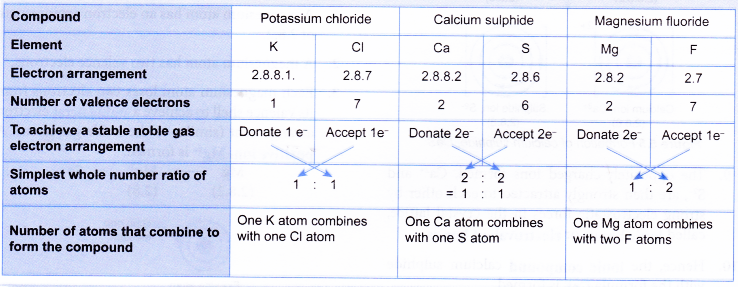Formation of potassium chloride, KCl

• A potassium atom has a proton number of 19 and an electron arrangement of 2.8.8.1.
• A potassium atom has one valence electron.
• During bond formation, each potassium atom loses one electron from its valence shell to achieve a stable octet electron arrangement (similar to the noble gas argon). A positive ion, K+ is formed.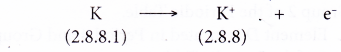• The electron lost by the potassium atom is transferred to a chlorine atom.
• A chlorine atom has a proton number of 17 and an electron arrangement of 2.8.7.
• A chlorine atom has seven valence electrons.
• Each chlorine atom gains one electron from a potassium atom into its valence shell to achieve a stable octet electron arrangement (similar to the noble gas argon). A negative ion. Cl is formed.• Figure shows the transfer of one electron from a potassium atom to a chlorine atom to form the ionic compound potassium chloride.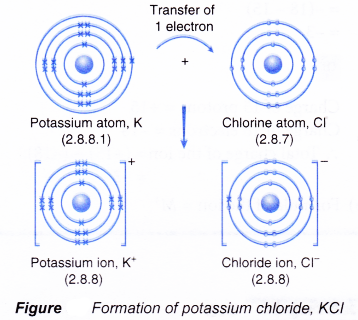• The oppositely-charged ions formed, K+ and Cl, are then strongly attracted to each other by strong electrostatic forces in the crystal lattice, called ionic bonds or electrovalent bonds.
• Hence, the ionic compound potassium chloride with the formula KCl is formed.

Formation of calcium sulphide, CaS

• A calcium atom has a proton number of 20 and an electron arrangement of 2.8.8.2.
• A calcium atom has two valence electrons.
• During bond formation, each calcium atom loses two electrons from its valence shell to achieve a stable octet electron arrangement (similar to the noble gas argon). A positive ion, Ca2+ is formed.• The two electrons lost by the calcium atom are transferred to the sulphur atom.
• A sulphur atom has a proton number of 16 and an electron arrangement of 2.8.6.
• A sulphur atom has six valence electrons.
• Each sulphur atom accepts two electrons from a calcium atom into its valence shell to achieve a stable octet electron arrangement (similar to the noble gas argon). A negative ion, S2- is formed.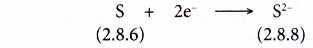• Figure shows the transfer of electrons from a calcium atom to a sulphur atom to form the ionic compound calcium sulphide.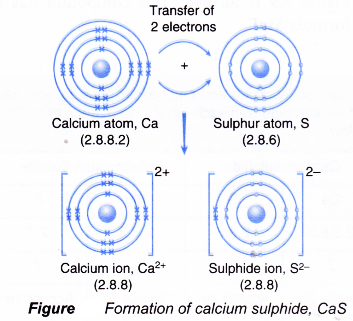• The oppositely-charged ions formed, Ca2+ and S2-, are then strongly attracted to each other by strong electrostatic forces in the crystal lattice, called ionic bonds or electrovalent bonds.
• Hence, the ionic compound calcium sulphide with the formula CaS is formed.

Formation of magnesium fluoride, MgF• A magnesium atom has an electron arrangement of 2.8.2.
• A magnesium atom has two valence electrons.
• Each magnesium atom loses two electrons from its valence shell to achieve a stable octet electron arrangement (similar to the noble gas neon). A positive ion, Mg2+ is formed.• A fluorine atom has an electron arrangement of 2.7.
• A fluorine atom has seven valence electrons.
• Each fluorine atom accepts one electron into its valence shell to achieve a stable octet electron arrangement (similar to the noble gas neon). A negative ion, F is formed.• During bond formation:
• One magnesium atom combines with two fluorine atoms.
• The magnesium atom loses two electrons from its valence shell.
• These two electrons are transferred to two fluorine atoms.
• Each of the two fluorine atoms accepts one electron from a magnesium atom.
• Hence, one magnesium ion, Mg2+ and two fluoride ions, F, are formed.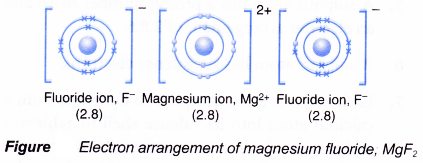• The oppositely-charged ions formed, Mg2+ and F, are then strongly attracted to each other by strong electrostatic forces in the crystal lattice, called ionic bonds or electrovalent bonds.
• Hence, the ionic compound magnesium fluoride with the electron arrangement as shown in above figure is formed. This compound has the formula MgF2.